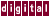## 7.3.8 Loop Fusion

Loop fusion is a conventional compiler optimization that transforms two adjacent loops into a single loop. The use of data dependence tests allows fusion of more loops than is possible with standard techniques. The `/scalaropt=2` qualifier or the `/optimize=5` qualifier is required to enable loop fusion.

In the following example, the first two loops can be fused and optimized together. Fusing these loops reduces `for` -loop overhead and the amount of synchronization required, thereby improving efficiency and speed. KAP recognizes that the third loop must be executed after the first two and does not fuse it with the others, as shown in the following example:

```for ( i=0; i<n; i++ ) {
a[i] = b[i] + c[i];
}

for ( i=0; i<n; i++ ) {
a[i] = a[i] + d[i];
}

for ( i=0; i<n; i++ ) {
d[i] = a[i+1];
}
```

Becomes:

```for ( i1 = 0; i1<n; i1++ ) {
a[i1] = b[i1] + c[i1];
a[i1] +=  d[i1];
}
for ( i = 0; i<n; i++ ) {
d[i] = a[i+1];
}
}
```

Previous Page Next Page Contents Index
Command-Line Qualifiers